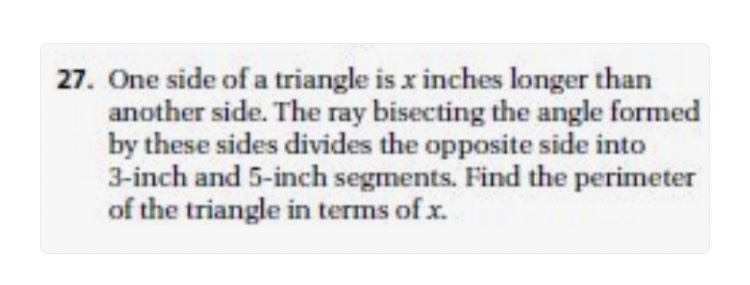### ¿Todavía tienes preguntas de matemáticas?

Pregunte a nuestros tutores expertos
Algebra
Pregunta27. One side of a triangle is $$x$$ inches longer than another side. The ray bisecting the angle formed by these sides divides the opposite side into 3-inch and $$5$$ -inch segments. Find the perimeter of the triangle in terms of $$x$$ .